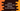# Python program to find out numbers in a list divisible by two numbers## Introduction :

In this python programming tutorial, we will learn how to find all numbers that are divisible by two specific numbers. For example, let’s take a look at the list_ [1,2,3,4,5]. In this list, numbers that are divisible by _2 and 1 are [2,4]. Our program will do the same thing. The user will enter the values of the list and also the two numbers (let’s say m and n).

This is a beginner friendly program and it will give you the basic understanding of loops, conditions, list and how to take user input in python. Let’s take a look at the program first :

### Python program :

``````#1
list_size = int(input("How many numbers are in the list : "))
#2
number_list = []
final_list = []
#3
for i in range(0,list_size):
number_list.append(int(input("Enter list item {} : ".format(i))))
#4
m = int(input("Enter the first divider : "))
n = int(input("Enter the second divider : "))
#5
for i in range(0,list_size):
if number_list[i] % m == 0 and number_list[i] % n == 0 :
final_list.append(number_list[i])
#6
print("Numbers that are divisible by {} and {} is : ".format(m,n),final_list)``````

### Explanation :

The commented numbers in the above program denote the step number below :

1. Ask the user how many numbers the list will hold. Read the input and store it in listsize_ variable. We are using ‘input()’ method to take the list size as an input from the user. We are wrapping this value with ‘int()’ to read the value as an integer from the user.
2. Create two empty lists. numberlist_ will hold all the numbers and resultlist_ will hold the final list of numbers. resultlist_ will hold the final list items and numberlist_ will hold all the numbers in the beginning.
3. Run one for loop. Ask the user to enter the list items one by one. Read all values and append to the numberlist_. We are using one for loop but you can also use one while loop if you want.
4. Ask the user to enter the value for m and n. Read both values and store them in variable m and n. Our goal is to find out all values of numberlist_ that are divisible by ‘m’ and ’n’.
5. For filtering out the required values from the list, we need to iterate through them all. Run one more for loop. Check for each number if it is divisible by m and n or not. If yes, add that value to the finallist. So, _finallist_ will store all the result numbers after the for loop will exit. For checking if a number is divisible by m and n or not, we are using ‘and’ operation. This operation will return True if both conditions before and after ‘and’ is True. Here, it will be True if the number is divisible by both ‘m’ and ’n’.
6. Finally, print out all the numbers that are in the finallist_.

### Conclusion :

We have learned how to find all numbers in a list divisible by m or n in python. This program taught you how to read user inputs, print outputs to the user, how to create an empty list and fill that list using user provided values, and how to filter out list items with a specific condition. Try to run the program by downloading the code using the list provided above.Go through the above example and drop a comment below if you have any queries. Happy Coding 🙂